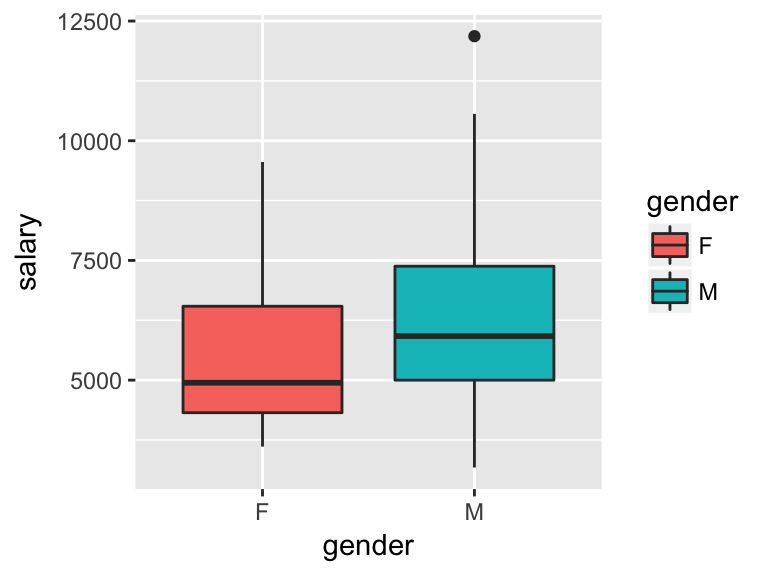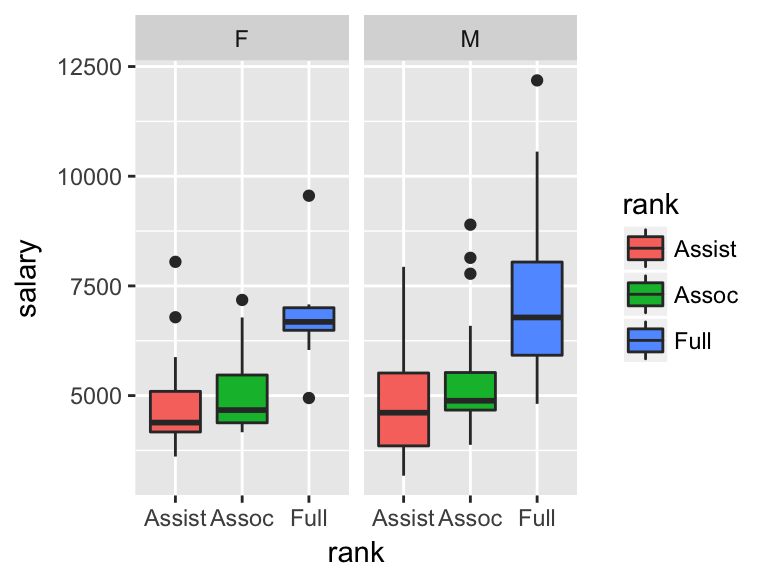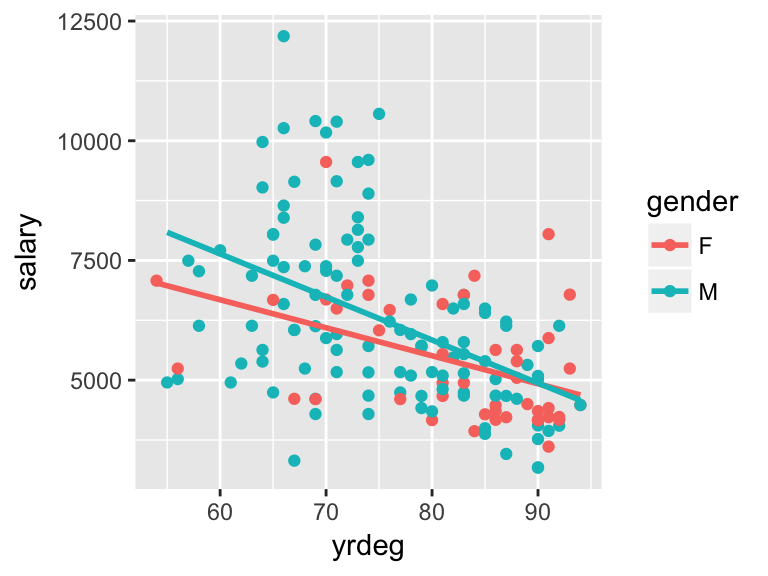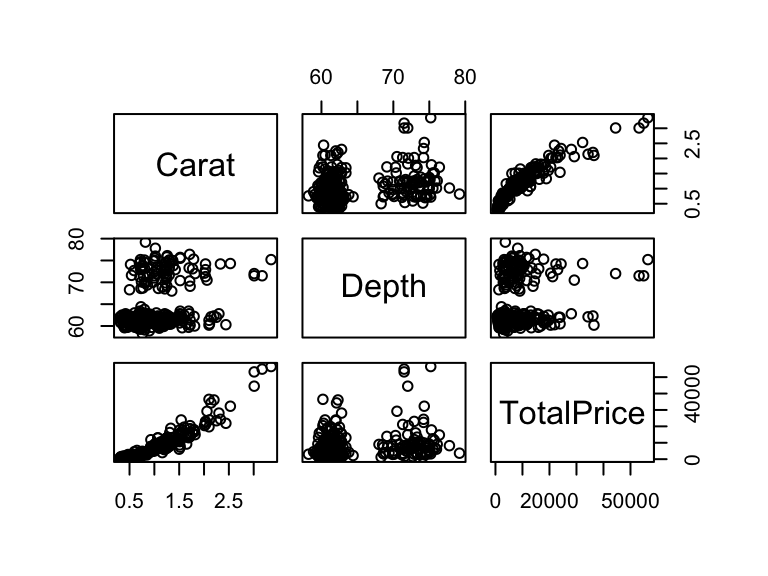Is there any evidence suggestive of discrimination by sex in the employment of the faculty at a University? Salary data was obtained on 159 faculty members employed by the University during the 1995 academic year. Along with the 1995 salary the following additional variables were also collected:

id = The anonymous identification number for the faculty member

gender = Gender of the faculty member (coded as M or F)

deg = The highest degree obtained by the faculty member (PhD, Professional, Other)

yrdeg = Year highest degree was obtained

field = Field of research during 1995 (Arts, Professional, Other)

startyr = Year starting employment at the university

year = Year of data collection (1995 for all)

rank = Faculty rank as of 1995 (Assistant, Associate, Full)

admin = Does faculty member hold an administrative position as of 1995 = (0=No, 1=Yes)

salary = 1995 salary in US dollars

First, let’s get a quick look at the data. Other than our predictor of interest, gender, think about which predictors might be useful in explaining salary- i.e. might be confounding variables.

summary(salary)
##        id         gender     deg          yrdeg         field
##  Min.   :   6.0   F: 47   Other:  8   Min.   :54.00   Arts : 24
##  1st Qu.: 453.0   M:112   PhD  :135   1st Qu.:69.00   Other:112
##  Median : 931.0           Prof : 16   Median :78.00   Prof : 23
##  Mean   : 904.5                       Mean   :77.18
##  3rd Qu.:1324.5                       3rd Qu.:86.00
##  Max.   :1765.0                       Max.   :94.00
##     startyr           year        rank        admin            salary
##  Min.   :61.00   Min.   :95   Assist:39   Min.   :0.0000   Min.   : 3175
##  1st Qu.:73.00   1st Qu.:95   Assoc :45   1st Qu.:0.0000   1st Qu.: 4670
##  Median :84.00   Median :95   Full  :75   Median :0.0000   Median : 5691
##  Mean   :81.62   Mean   :95               Mean   :0.1195   Mean   : 6013
##  3rd Qu.:90.00   3rd Qu.:95               3rd Qu.:0.0000   3rd Qu.: 6977
##  Max.   :95.00   Max.   :95               Max.   :1.0000   Max.   :12184
ggplot(salary) + geom_boxplot(aes(x = gender, y = salary, fill = gender))ggplot(salary) + geom_boxplot(aes(x = rank, y = salary, fill = rank)) +
facet_wrap(~gender)ggplot(salary, aes(x = yrdeg, y = salary, col = gender)) + geom_point() + geom_smooth(method = "lm", se = FALSE)table(salary$rank, salary$gender) 
##
##           F  M
##   Assist 20 19
##   Assoc  15 30
##   Full   12 63

### Correlation Matrix

In order to get a good idea of how quantitative variables are correlated with each other, we can create a correlation matrix. Note: this data set only has 3 continuous variables, so this will be a rather small matrix since we can’t include factors.

cor(salary[, c("yrdeg","startyr", "salary")])
##              yrdeg    startyr     salary
## yrdeg    1.0000000  0.8313906 -0.4910319
## startyr  0.8313906  1.0000000 -0.2984846
## salary  -0.4910319 -0.2984846  1.0000000

### Nested F tests

Let’s look at a variety of models with gender and starting year as our two predictors.

Side note, it is good practice in statistics to name indicator variables by the name of the variable when the indicator is equal to 1. Instead of gender, let’s code it male where male = 1 indicates a male and male = 0 indicates a female. This way you don’t forget which one is 0 or 1.

salary$male <- factor(salary$gender, levels = c("F","M"), labels = c(0,1))
summary(salary[, c("gender", "male")]) # Double check
##  gender  male
##  F: 47   0: 47
##  M:112   1:112

Mod1: $$Y = \beta_0 + \epsilon$$

Mod2: $$Y = \beta_0 + \beta_1 \ male + \epsilon$$

Mod3: $$Y = \beta_0 + \beta_1 \ yrdeg + \epsilon$$

Mod4: $$Y = \beta_0 + \beta_1 \ male + \beta_2 \ yrdeg + \epsilon$$

Mod5: $$Y = \beta_0 + \beta_1 \ yrdeg + \beta_2 \ male + \epsilon$$

Mod6: $$Y = \beta_0 + \beta_1 \ male + \beta_2 \ yrdeg + \beta_3 \ male*yrdeg + \epsilon$$

Mod7: $$Y = \beta_0 + \beta_1 \ yrdeg + \beta_2 \ male + \beta_3 \ male*yrdeg + \epsilon$$

mod1 <- lm(salary ~ 1, data = salary)
mod2 <- lm(salary ~ male, data = salary)
mod3 <- lm(salary ~ yrdeg, data = salary)
mod4 <- lm(salary ~ male + yrdeg, data = salary)
mod5 <- lm(salary ~ yrdeg + male, data = salary)
mod6 <- lm(salary ~ male*yrdeg, data = salary)
mod7 <- lm(salary ~ yrdeg*male, data = salary)

Nested F-test = t-test = Overall F-tests = Correlation Test

anova(mod1, mod3) 
## Analysis of Variance Table
##
## Model 1: salary ~ 1
## Model 2: salary ~ yrdeg
##   Res.Df       RSS Df Sum of Sq      F    Pr(>F)
## 1    158 480585503
## 2    157 364710416  1 115875087 49.882 4.988e-11 ***
## ---
## Signif. codes:  0 '***' 0.001 '**' 0.01 '*' 0.05 '.' 0.1 ' ' 1
summary(mod3) 
##
## Call:
## lm(formula = salary ~ yrdeg, data = salary)
##
## Residuals:
##     Min      1Q  Median      3Q     Max
## -3561.3  -907.7  -158.7   843.6  5219.6
##
## Coefficients:
##             Estimate Std. Error t value Pr(>|t|)
## (Intercept) 12579.70     937.60  13.417  < 2e-16 ***
## yrdeg         -85.08      12.05  -7.063 4.99e-11 ***
## ---
## Signif. codes:  0 '***' 0.001 '**' 0.01 '*' 0.05 '.' 0.1 ' ' 1
##
## Residual standard error: 1524 on 157 degrees of freedom
## Multiple R-squared:  0.2411, Adjusted R-squared:  0.2363
## F-statistic: 49.88 on 1 and 157 DF,  p-value: 4.988e-11
cor.test(salary$yrdeg, salary$salary)
##
##  Pearson's product-moment correlation
##
## data:  salary$yrdeg and salary$salary
## t = -7.0627, df = 157, p-value = 4.988e-11
## alternative hypothesis: true correlation is not equal to 0
## 95 percent confidence interval:
##  -0.6007641 -0.3631385
## sample estimates:
##        cor
## -0.4910319

Nested F-tests = t-test (for yrdeg)

anova(mod2, mod4) 
## Analysis of Variance Table
##
## Model 1: salary ~ male
## Model 2: salary ~ male + yrdeg
##   Res.Df       RSS Df Sum of Sq      F    Pr(>F)
## 1    157 454628083
## 2    156 361439784  1  93188299 40.221 2.335e-09 ***
## ---
## Signif. codes:  0 '***' 0.001 '**' 0.01 '*' 0.05 '.' 0.1 ' ' 1
summary(mod4) 
##
## Call:
## lm(formula = salary ~ male + yrdeg, data = salary)
##
## Residuals:
##     Min      1Q  Median      3Q     Max
## -3611.1  -953.8  -251.7   796.9  5174.6
##
## Coefficients:
##             Estimate Std. Error t value Pr(>|t|)
## (Intercept) 11981.94    1062.98  11.272  < 2e-16 ***
## male1         331.03     278.61   1.188    0.237
## yrdeg         -80.36      12.67  -6.342 2.34e-09 ***
## ---
## Signif. codes:  0 '***' 0.001 '**' 0.01 '*' 0.05 '.' 0.1 ' ' 1
##
## Residual standard error: 1522 on 156 degrees of freedom
## Multiple R-squared:  0.2479, Adjusted R-squared:  0.2383
## F-statistic: 25.71 on 2 and 156 DF,  p-value: 2.232e-10

Why is the overall F-test different here? What are the null and alternative hypotheses?

Nested F-tests = t-tests $$\neq$$ Overall F-test.

anova(mod1, mod4)
## Analysis of Variance Table
##
## Model 1: salary ~ 1
## Model 2: salary ~ male + yrdeg
##   Res.Df       RSS Df Sum of Sq      F    Pr(>F)
## 1    158 480585503
## 2    156 361439784  2 119145719 25.712 2.232e-10 ***
## ---
## Signif. codes:  0 '***' 0.001 '**' 0.01 '*' 0.05 '.' 0.1 ' ' 1
summary(mod4) 
##
## Call:
## lm(formula = salary ~ male + yrdeg, data = salary)
##
## Residuals:
##     Min      1Q  Median      3Q     Max
## -3611.1  -953.8  -251.7   796.9  5174.6
##
## Coefficients:
##             Estimate Std. Error t value Pr(>|t|)
## (Intercept) 11981.94    1062.98  11.272  < 2e-16 ***
## male1         331.03     278.61   1.188    0.237
## yrdeg         -80.36      12.67  -6.342 2.34e-09 ***
## ---
## Signif. codes:  0 '***' 0.001 '**' 0.01 '*' 0.05 '.' 0.1 ' ' 1
##
## Residual standard error: 1522 on 156 degrees of freedom
## Multiple R-squared:  0.2479, Adjusted R-squared:  0.2383
## F-statistic: 25.71 on 2 and 156 DF,  p-value: 2.232e-10

When only the nested F-test applies. What are we testing?

anova(mod2, mod7)
## Analysis of Variance Table
##
## Model 1: salary ~ male
## Model 2: salary ~ yrdeg * male
##   Res.Df       RSS Df Sum of Sq      F    Pr(>F)
## 1    157 454628083
## 2    155 358408647  2  96219435 20.806 9.908e-09 ***
## ---
## Signif. codes:  0 '***' 0.001 '**' 0.01 '*' 0.05 '.' 0.1 ' ' 1

When the order matters in a nested F-test.

anova(mod4); summary(mod4)
## Analysis of Variance Table
##
## Response: salary
##            Df    Sum Sq  Mean Sq F value    Pr(>F)
## male        1  25957420 25957420  11.203  0.001023 **
## yrdeg       1  93188299 93188299  40.221 2.335e-09 ***
## Residuals 156 361439784  2316922
## ---
## Signif. codes:  0 '***' 0.001 '**' 0.01 '*' 0.05 '.' 0.1 ' ' 1
##
## Call:
## lm(formula = salary ~ male + yrdeg, data = salary)
##
## Residuals:
##     Min      1Q  Median      3Q     Max
## -3611.1  -953.8  -251.7   796.9  5174.6
##
## Coefficients:
##             Estimate Std. Error t value Pr(>|t|)
## (Intercept) 11981.94    1062.98  11.272  < 2e-16 ***
## male1         331.03     278.61   1.188    0.237
## yrdeg         -80.36      12.67  -6.342 2.34e-09 ***
## ---
## Signif. codes:  0 '***' 0.001 '**' 0.01 '*' 0.05 '.' 0.1 ' ' 1
##
## Residual standard error: 1522 on 156 degrees of freedom
## Multiple R-squared:  0.2479, Adjusted R-squared:  0.2383
## F-statistic: 25.71 on 2 and 156 DF,  p-value: 2.232e-10
anova(mod5); summary(mod5)
## Analysis of Variance Table
##
## Response: salary
##            Df    Sum Sq   Mean Sq F value   Pr(>F)
## yrdeg       1 115875087 115875087 50.0125 4.83e-11 ***
## male        1   3270632   3270632  1.4116   0.2366
## Residuals 156 361439784   2316922
## ---
## Signif. codes:  0 '***' 0.001 '**' 0.01 '*' 0.05 '.' 0.1 ' ' 1
##
## Call:
## lm(formula = salary ~ yrdeg + male, data = salary)
##
## Residuals:
##     Min      1Q  Median      3Q     Max
## -3611.1  -953.8  -251.7   796.9  5174.6
##
## Coefficients:
##             Estimate Std. Error t value Pr(>|t|)
## (Intercept) 11981.94    1062.98  11.272  < 2e-16 ***
## yrdeg         -80.36      12.67  -6.342 2.34e-09 ***
## male1         331.03     278.61   1.188    0.237
## ---
## Signif. codes:  0 '***' 0.001 '**' 0.01 '*' 0.05 '.' 0.1 ' ' 1
##
## Residual standard error: 1522 on 156 degrees of freedom
## Multiple R-squared:  0.2479, Adjusted R-squared:  0.2383
## F-statistic: 25.71 on 2 and 156 DF,  p-value: 2.232e-10

Look at each line in the above ANOVA tables. Find it’s equalivalent t-test and/ or overall F-test using the models described above.

Also, in both summaries for mod4 and mod5 we get the same p-value for the t-test of male as the sequential F-test for male from anova(mod5), but not anova(mod4). Why does this happen?

Testing for an interaction. Will order matter here?

anova(mod4, mod6) 
## Analysis of Variance Table
##
## Model 1: salary ~ male + yrdeg
## Model 2: salary ~ male * yrdeg
##   Res.Df       RSS Df Sum of Sq      F Pr(>F)
## 1    156 361439784
## 2    155 358408647  1   3031137 1.3109  0.254
anova(mod5, mod6) 
## Analysis of Variance Table
##
## Model 1: salary ~ yrdeg + male
## Model 2: salary ~ male * yrdeg
##   Res.Df       RSS Df Sum of Sq      F Pr(>F)
## 1    156 361439784
## 2    155 358408647  1   3031137 1.3109  0.254

Interpret each coefficient in Model 6 in context of the problem.

### Indicator Variables When We Have More Than Two Categories

Up until now you’ve mostly been using indicators for binary variables. As you can see from this data set, there are many other variables with 3 categories that could be extremely useful in our model.

mod7 <- lm(salary ~ male + yrdeg + rank, data = salary)
summary(mod7)
##
## Call:
## lm(formula = salary ~ male + yrdeg + rank, data = salary)
##
## Residuals:
##     Min      1Q  Median      3Q     Max
## -2503.1  -867.8  -297.9   467.9  4946.9
##
## Coefficients:
##             Estimate Std. Error t value Pr(>|t|)
## (Intercept)  6425.93    1455.38   4.415 1.89e-05 ***
## male1         164.29     255.57   0.643    0.521
## yrdeg         -19.72      16.13  -1.222    0.223
## rankAssoc     262.92     329.96   0.797    0.427
## rankFull     1948.61     397.61   4.901 2.40e-06 ***
## ---
## Signif. codes:  0 '***' 0.001 '**' 0.01 '*' 0.05 '.' 0.1 ' ' 1
##
## Residual standard error: 1384 on 154 degrees of freedom
## Multiple R-squared:  0.3864, Adjusted R-squared:  0.3704
## F-statistic: 24.24 on 4 and 154 DF,  p-value: 1.434e-15

Similar to how you have two levels in an explanatory where only one level shows up in the model, with more than two levels one level will also not show up in the model. We call this one level, the reference. When interpreting coefficients you are comparing back to the reference.

For example: $$\hat{\beta}_3$$ is the predicted change in salary for Associate professors compared to Assistant professors who are similar in gender and the year they got their highest degree.

### Squaring Predictors in a Model

Note, this example comes from the textbook starting on page 120.

library(Stat2Data)
data('Diamonds')
plot(Diamonds[, c(1, 4, 6)])ggplot(Diamonds) + geom_point(aes(x = Carat, y = TotalPrice))